## Thursday, March 31, 2022

### Will inflation persist?

(Note: this post uses Mathjax equations. If you see garbage, come back to the original.)

Introduction

Will inflation persist? One line of thought says no: This inflation came from a one-time fiscal blowout. That "stimulus'' being over, inflation should stop. In fiscal language, we had a one-time big deficit, that people do not expect to be repaid by future surpluses. That gives rise to a one-time price-level increase, paying for the deficit by inflating away some debt, but then it's over.

There are many objections to this argument: We still have persistent deficits, and the entitlement deluge is coming. Or, maybe our inflation comes from something else.

Here, I analyze one simple point. Suppose we do, in fact, have a one-time large deficit. How much do sticky prices and policy responses draw a one-time deficit shock out to a long-lasting inflation? The answer is, quite a bit. (This post is an extension of "Fiscal Inflation," which documents the size and nature of the fiscal shock to inflation, and talks through the frictionless model.)

Frictionless case

Start with the simplest new-Keynesian model \begin{align} i_t & = E_t \pi_{t+1} \label{fish} \\ \rho v_{t+1} &= v_t + i_t - \pi_{t+1} - \tilde{s}_{t+1}. \label{vt} \end{align}

The variable $$v$$ is the real value of government debt, $$\rho=e^{-r}$$ is a constant slightly less than one, and $$\tilde{s}$$ is the real primary surplus scaled by the value of debt. Equation (\ref{vt}) is a linearized version of the debt accumulation equation: The real value of debt increases by the real interest rate or interest costs of the debt, and decreases by any primary surpluses.

I analyze a shock to surpluses $$\tilde{s}_t$$, and I start by specifying no change to the interest rate $$i_t$$. That has been the case for a year, and the responses the Fed is announcing so far are so much smaller than inflation, that it's not a bad approximation in general. I'll add a stronger monetary policy response later.

We can Iterate forward (\ref{vt}) , take $$\Delta E_{t+1}\equiv E_{t+1}-E_t$$ of both sides, use (\ref{fish}), to get \begin{equation} \Delta E_{t+1} \pi_{t+1} = -\Delta E_{t+1} \sum_{j=0}^\infty \rho^j \tilde{s}_{t+1+j} = -\varepsilon_{s,t+1}. \label{Depi} \end{equation} Unexpected inflation is the revision in the present value of surpluses. Thus, the solutions of this model are \begin{align*} i_t & = E_t \pi_{t+1} \\ \Delta E_{t+1} \pi_{t+1} & = -\varepsilon_{s,t+1} . \end{align*} The interest rate sets expected inflation; the fiscal shock drives unexpected inflation.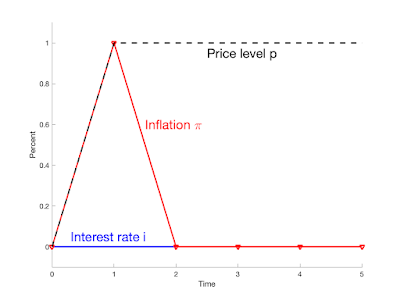Figure 1. Response to a deficit shock equal to 1% of outstanding debt. Frictionless model with no monetary policy response.

Figure 1 plots the response to a 1% deficit shock with no change in the interest rate. The result is a 1% unexpected price-level rise; a 1% transitory inflation.

If this is the model, and if this is the shock, inflation will soon end. We only have to decide what a "period'' is.

Now, we had a 30% shock; $$\tilde{s}$$ is surplus divided by the value of debt. The $5 trillion cumulative deficit was almost 30% of the$17 trillion in debt outstanding at the beginning of the pandemic. We have seen only about 8% inflation so far. But the inflationary fiscal shock is the shock to the discounted sum of deficits and surpluses in (\ref{Depi}), not just the shock to today's deficit. (This is a prime way in which a fiscal analysis differs from traditional Keynesian multiplier + gap analysis.)

So, if people expect most of the deficit to be repaid; if the 30% deficit ($$s_1$$) shock comes with 22% rise in future surpluses $$s_{1+j}$$, then we have only an 8% shock to the discounted stream of surpluses $$\varepsilon_s$$, resulting in the 8% price-level rise we have just seen. And it's over, at least until there is another shock to deficits, to people's expectations about that future partial repayment, or to monetary policy.

While I think of this system in terms of fiscal theory, the same analysis applies if you think in strictly new-Keynesian terms. We observe the surplus and interest rate, so our question is what happens to equilibrium inflation given those paths. Who was active vs. passive in getting to that surplus and interest rate doesn't matter. You may view the situation that the Federal Reserve woke up and proclaimed a set of monetary policy disturbances and an active Taylor rule, that result in a sharp inflation though no change in observed interest rate---the $$\phi \pi_t$$ just offset the $$u_t$$ in $$i_t = \phi \pi_t + u_t$$. (To calculate such a policy, first write the rule $$i_t = i^\ast_t + \phi(\pi_t - \pi^\ast_t)$$. Choose the $$i_t^\ast$$ and $$\pi_t^\ast$$ you want to see, then rewrite the rule with $$u_t = i^\ast_t - \phi\pi^\ast_t$$.) Then, fiscal policy "passively'' accomodated this inflation with a large deficit. You may read (\ref{Depi}) as a calculation of the "passive'' fiscal consequences of such a Fed-chosen inflation. A big point of Fiscal Theory of the Price Level is that for many purposes, such as this one, one can be agnostic about equilibrium selection while analyzing monetary--fiscal interactions.

Sticky Prices

But prices are sticky, and sticky prices may draw out the inflation response to a fiscal shock.

I use the most standard new-Keynesian model: \begin{align} x_{t} & =E_{t}x_{t+1}-\sigma(i_{t}-E_{t}\pi_{t+1})\label{equil1}\\ \pi_{t} & =\beta E_{t}\pi_{t+1}+\kappa x_{t} \label{equil2}\\ \rho v_{t+1} & =v_{t}+i_t-\pi_{t+1}-\tilde{s}_{t+1}.\label{equil3} \end{align}

The response of this model to a unit deficit shock at time 1 is given by \begin{equation} \pi_t= (1-\rho\lambda_1^{-1})\lambda_1^{-(t-1)}, \label{lambda} \end{equation} where $\lambda_1 = \left[ \left(1+\beta+\sigma\kappa\right) + \sqrt{\left(1+\beta+\sigma\kappa\right)^2-4\beta}\right]/2.$ (Details below.)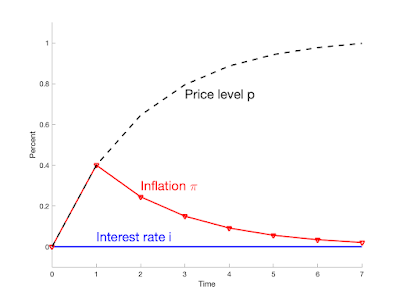Figure 2. Response to a deficit shock equal to 1% of outstanding debt. Sticky prices with no monetary policy response. Parameters $$\sigma = 1$$, $$\kappa = 0.25$$, $$\beta=0.99$$, $$\rho = 0.98$$.

Figure 2 presents the response of inflation to the same deficit shock, with no interest rate response, with sticky prices. Sticky prices draw out the inflation response.

The total rise in the price level is the same, 1.0, but it is spread over time. In essence, the frictionless model of Figure 1 does describe the fiscal shock, but a "period'' is 7 years.

If this graph is right, we have a good deal of inflation left to go. The first year only produces about 40% of the total eventual price level rise. In this interpretation, people do not expect the majority of the 5 trillion deficit, 30% of debt to be repaid. Of course, the conclusion depends on parameters, and especially on the product of intertemporal substitution times price stickiness $$\sigma \kappa$$. If prices are much less sticky than I have specified, these dynamics might describe a period of two years, as the Fed seems currently to believe, or even just the smooth one year of inflation that we just experienced. But $$\kappa=0.25$$ is if anything less sticky than typical specifications. $$\kappa=0.25$$ means that a 1% GDP gap and steady expectations $$E_t \pi_{t+1}$$ implies 0.25% inflation; by Okun's law a 1 percentage point decline in unemployment implies 0.5% inflation. This is if anything a good deal steeper, less sticky, Phillips curve than most studies find. With price stickiness, the fundamental story changes. In a frictionless model, we digest the story simply: Unexpected inflation, an unexpected one-time price level increase, lowers the real value of outstanding debt, just as would a partial default. But this model still maintains one-period debt, so a slow expected inflation cannot devalue debt. Instead, in this model, there is a long period of negative real interest rates---as we are observing in reality. This period of negative real interest rates slowly lowers the real value of government debt. With sticky prices, even short-term bondholders cannot escape. The initial inflation is much lower with sticky prices. How does this happen in light of (\ref{Depi})? From (\ref{equil3}), in this model we generalize (\ref{Depi}) have \begin{equation} \Delta E_{t+1} \pi_{t+1} = -\Delta E_{t+1} \sum_{j=0}^\infty \rho^j \tilde{s}_{t+1+j} + \sum_{j=1}^\infty \rho^j (i_{t+j}-\pi_{t+1+j} ). \label{identity} \end{equation} The second term is a discount rate term. Higher real interest rates are a lower discount factor for government surpluses and lower the value of debt, an inflationary force. Equivalently, higher real interest rates are a higher interest cost of the debt, that acts just like greater deficits to induce (or follow, for you new-Keynesians) inflation. That price stickiness draws out the inflationary response to a fiscal shock is perhaps not that surprising. Many stories feature such stickiness, and suggest substantial inflationary momentum. Price hikes take time to work through to wages, which then lead to additional price hikes. Housing prices take time to feed in to rents. Input price rises take time to lead to output price rises. But such common stories reflect an idea of backward looking price stickiness. The Phillips curve in (\ref{equil2}) is entirely forward looking. Inflation is a jump variable. Indeed, in the standard new-Kenesian solutions, inflation can rise instantly and permanently in response to a permanent monetary policy shock, with no dynamics at all. (Add $$i_t = \phi \pi_t + u_t$$, $$u_t = 1.0 u_{t-1} + \varepsilon_{i,t}$$. Inflation and interest rates move equally, instantly and permanently to the shock.) One might well add such backward looking terms, e.g. $\pi_t = \alpha \pi_{t-1} + \beta E_t \pi_{t+1} + \kappa x_t$ and such terms are often used. It seems natural that such terms would only spread out even further the inflation response to the fiscal shock, but that conjecture needs to be checked. Sticky Prices and Monetary Policy What if the Fed does eventually respond? To address that question, I add an interest rate policy rule and long-term debt to the model. The model is \begin{align} x_{t} & =E_{t}x_{t+1}-\sigma(i_{t}-E_{t}\pi_{t+1})\label{ISvstar}\\ \pi_{t} & =\beta E_{t}\pi_{t+1}+\kappa x_{t}\label{NKvstar}\\ i_{t} & =\theta_{i\pi}\pi_{t}+\theta_{ix}x_{t}\label{nm4}\\ \rho v_{t+1} & =v_{t}+r_{t+1}^{n}-\pi_{t+1}-\tilde{s}_{t+1}\label{nm7}\\ E_{t}r_{t+1}^{n} & =i_{t}\label{nm8}\\ r_{t+1}^{n} & =\omega q_{t+1}-q_{t}.\label{nm9} \end{align} This is a simplified version of the model in Fiscal Theory of the Price Level Section 5.5. I turn off all the fiscal policy in that model, since the game here is to see what happens to inflation given the path of surpluses. The variable $$r^n_{t+1}$$ is the nominal return on the portfolio of all government bonds. Equation (\ref{nm8}) imposes the expectations hypothesis. Equation (\ref{nm9}) relates the return of the government debt portfolio to the change in its price, where $$\omega$$ describes a geometric term structure of debt. The face value of maturity $$j$$ debt declines at rate $$\omega^j$$.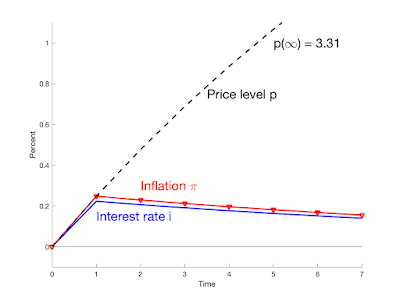Figure 3. Response to a 1% deficit shock, with a monetary policy rule. Parameters $$\sigma = 1$$, $$\kappa = 0.25$$, $$\beta = 0.99$$, $$\theta_\pi=0.9$$, $$\theta_x=0$$, $$\rho = 0.98$$, $$\omega=0.8$$. Figure 3 presents the response of this model. (Calculation below.) The interest rate now rises, with $$\theta_{\pi}$$ slightly less than one to a point just below the inflation rate. The effect of this monetary policy response is to reduce the initial inflation impact, from about 0.4% to 0.25%, and to further smooth inflation over time. If this is our world, we are only beginning to see the inflationary response to our one-time fiscal shock! The mechanism captures one aspect of current intuition: By raising interest rates, the Fed lowers near-term inflation from what it otherwise would be. An interest rate rise in this model lowers inflation in the near term. But this model captures a form of unpleasant arithmetic. There has been a fiscal shock, a deficit that will not be repaid, and at some point some debt must be inflated away as a result of that deficit. Monetary policy here can shift inflation around over time, but monetary policy cannot eliminate inflation entirely. Thus, the lower initial inflation is paid for by raising later inflation. Monetary policy smooths the inflation response, but cannot eliminate it entirely. In fact, the cumulative inflation in this model is 3.38%, three times larger than the 1% cumulative inflation of the last two models. The Fed in this simulation spreads inflation forward to fall more heavily on long-term bond holders, whose claims are devalued when they come due, and thereby lightens the load on short-term bondholders, who do not experience much inflation. But the mechanical rule spreads inflation forward even further than that, as the maturity structure of the debt, with coefficient 0.8 is shorter than this inflation response. A more sophisticated rule could achieve the same reduction in current inflation with a smaller total price-level rise. For now, if this is our world, not only will we see the nearly 30% total price level rise suggested by the previous model, we will see a total price level rise nearly three times greater. Update: The last scenario doesn't give the Fed much power. But moving inflation to the future might also give some breathing space for fiscal policy to reverse, for Congress and administration to wake up and solve the long-run budget problem, and give an opposite fiscal shock. They don't seem in much mood to do so at the moment, but if inflation persists and its fiscal roots become more apparent, that mood may change. The next post in the series: Is the Fed Fisherian? Calculations To derive (\ref{lambda}), eliminate $$x_t$$ from (\ref{equil1})-(\ref{equil2}). Then express inflation as a two-sided moving average of the interest rate, plus a transient, as given by Fiscal Theory of the Price Level Appendix Section A1.5, equation (A1.47). With interest rates stuck at zero, all that is left is the transient. With the shock at time 1, it is $\pi_t = \pi_1 \lambda_1^{-(t-1)}$ Iterate forward (\ref{identity}) to find $$\pi_1$$. With no change in interest rate and the 1% deficit shock at time 1, (\ref{identity}) reduces to $\pi_{1} = 1- \sum_{j=1}^\infty \rho^j \pi_{1+j}$ $\sum_{j=0}^\infty \rho^j \pi_{1+j} = 1$ $\frac{1}{1-\rho\lambda_1^{-1}} \pi_1= 1$ $\pi_1= 1-\rho\lambda_1^{-1}.$ To make Figure 3, I use the matrix solution method described in Fiscal Theory of the Price Level Section A1.5.2. We just need to express the model in standard form, $A z_{t+1} = B z_t + C \varepsilon_{t+1} + D \delta_{t+1}$ where $$\delta_{t+1}$$ is a vector of expectational errors, induced by the fact that model equations only specify expectations of some variables. Rewrite the model \begin{align*} E_{t}x_{t+1}+\sigma E_{t}\pi_{t+1} & =x_{t}+\sigma\left( \theta_{\pi}% \pi_{t} + \theta_x x_t \right) \\ \beta E_{t}\pi_{t+1} & =\pi_{t}-\kappa x_{t}\\ \rho v_{t+1}+\pi_{t+1} -\omega q_{t+1}& =v_{t}-q_{t}-\tilde{s}_{t+1}\\ \omega E_t q_{t+1} & = q_t + \theta_{\pi}\pi_t + \theta_x x_t. \end{align*} In matrices $\left[ \begin{array} [c]{ccccc}% 1 & \sigma & 0 & 0 \\ 0 & \beta & 0 & 0 \\ 0 & 1 & \rho & -\omega\\ 0 & 0 & 0 & \omega \end{array} \right] \left[ \begin{array} [c]{c}% x_{t+1}\\ \pi_{t+1}\\ v_{t+1}\\ q_{t+1} \end{array} \right] =\left[ \begin{array} [c]{ccccc}% 1+\sigma\theta_x & \sigma\theta_{\pi} & 0 & 0\\ -\kappa & 1 & 0 & 0 \\ 0 & 0 & 1 & -1 \\ \theta_x & \theta_\pi & 0 & 1\\ \end{array} \right] \left[ \begin{array} [c]{c}% x_{t}\\ \pi_{t}\\ v_{t}\\ q_{t} \end{array} \right]$% $-\left[ \begin{array} [c]{c}% 0 \\ 0 \\ 1 \\ 0 \end{array} \right] s_{t+1} +\left[ \begin{array} [c]{ccc}% 1 & \sigma & 0\\ 0 & \beta & 0 \\ 0 & 0 & 0 \\ 0 & 0 & \omega\\ \end{array} \right] \left[ \begin{array} [c]{c}% \delta_{x,t+1}\\ \delta_{\pi,t+1}\\ \delta_{q,t+1}% \end{array} \right]$ Eigenvalue decompose $$A^-1B$$, and solve unstable roots forward and stable roots backward. Code Not very pretty. clear all close all sig = 1; kap = 0.25; bet = 0.99; thpi = 0.9; % verify with th = 0 gives same answer as simple thx = 0.0; rho = 0.98; omeg = 0.8; T = 100; show_results=1; % frictionless tim = (0:T)'; pit = 0*tim; pit(2) = 1; pt = cumsum(pit); figure; hold on; plot(tim, 0*tim,'-b','linewidth',2); plot(tim, pit,'-rv', 'linewidth',2); plot(tim, pt, '--k','linewidth',2); axis([0 5 -0.1 1.1]); xticks([0 1 2 3 4 5]); xlabel('Time'); ylabel('Percent'); text(1.5,0.6,'Inflation \pi','color','r','fontsize',18); text(0.3,0.05,'Interest rate i','color','b','fontsize',18); text(2.5,0.95,'Price level p','color','k','fontsize',18); print -depsc2 frictionless_inflation.eps; print -dpng frictionless_inflation.png; % simple price stickiness l1 = ((1+bet+sig*kap) + ((1+bet+sig*kap)^2-4*bet)^0.5)/2; pit2 = [0;(1/l1).^(tim(2:end)-1)]*(1-rho/l1); pt2 = cumsum(pit2); figure; hold on; plot(tim,0*tim,'-b','linewidth',2); plot(tim, pit2,'-rv', 'linewidth',2); plot(tim, pt2, '--k','linewidth',2); xlabel('Time'); ylabel('Percent'); axis([0 7 -0.1 1.1]); text(2,0.3,'Inflation \pi','color','r','fontsize',18); text(3,0.75,'Price level p','color','k','fontsize',18); text(1,0.05,'Interest rate i','color','b','fontsize',18); print -depsc2 sticky_inflation.eps; print -dpng sticky_inflation.png; % price stickiness and policy [xt pit vt qt it rnt pt] = solveit(sig,kap, bet, thpi, thx, rho, omeg,T); figure; hold on; plot(tim,it,'-b','linewidth',2); plot(tim, pit, '-rv','linewidth',2); plot(tim, pt, '--k','linewidth',2); plot(tim,0*tim,'-k'); xlabel('Time'); ylabel('Percent'); axis([0 7 -0.1 1.1]) text(2,0.3,'Inflation \pi','color','r','fontsize',18); text(3.5,0.75,'Price level p','color','k','fontsize',18); text(5,1,['p(\infty) = ' num2str(pt(end),'%4.2f')],'color','k','fontsize',18); text(1,0.15,'Interest rate i','color','b','fontsize',18); print -depsc2 policy_inflation.eps; print -dpng policy_inflation.png; disp('total inflation'); disp(pt(end)); %****** function [xt pit vt qt it rnt pt] = solveit(sig,kap, bet, thpi, thx, rho, omeg,T); show_results = 1; N = 4; A = [... 1 sig 0 0 ; 0 bet 0 0 ; 0 1 rho -omeg ; 0 0 0 omeg ]; % x pi q ui us B = [ ... 1+sig*thx sig*thpi 0 0 ; -kap 1 0 0 ; 0 0 1 -1 ; thx thpi 0 1 ]; C = [... 0 ; 0 ; 1 ; 0 ]; D = [... 1 sig 0 ; 0 bet 0 ; 0 0 0 ; 0 0 omeg ]; % Solve by eigenvalues taken from ftmp_model_final A1 = inv(A); F = A1*B; [Q L] = eig(F); Q1 = inv(Q); if show_results; disp('Eigenvalues'); disp(abs(diag(L)')); end % produce Ef, Eb, that select forward and backward % eigvenvalues. If L>=1 in position 1, 3, % produce % 1 0 0 0 % 0 0 1 0 ... % for example nf = find(abs(diag(L))>=1); % nf is the index of eigenvalues greater than one if show_results disp('number of eigenvalues >=1'); disp(size(nf,1)) end if (size(nf,1) < size(D,2)); disp('not enough eigenvalues greater than 1'); end; Ef = zeros(size(nf,1),size(A,2)); Efstar = zeros(size(A,2),size(A,2)); for indx = 1:size(nf,1); Ef(indx,nf(indx))=1; Efstar(nf(indx),nf(indx)) = 1; end; nb = find(abs(diag(L))<1); Eb = zeros(size(nb,1),size(A,2)); for indx = 1:size(nb,1); Eb(indx,nb(indx))=1; end; ze = Eb*Q1*A1*(C-D*inv(Ef*Q1*A1*D)*Ef*Q1*A1*C); % how epsilon shocks map to z. % in principle the forward z are zero. In practice they are 1E-16 and then % grow. So I go through the trouble of simulating forward only the nonzero % z and eigenvalues less than one. Nb = size(Eb,1); % number of stable z's Lb = (Eb*L*Eb'); % diagonal with only stable Ls % impulse response H = T+1; shock = 1; zbt = zeros(H,Nb); zbt(2,:) = (ze*shock)'; for t = 3:H; zbt(t,:) = Lb*zbt(t-1,:)'; end; % now create the full z with zeros zt = zeros(H,N); zt(:,nb) = zbt; % create original variables yt = zt*Q'; xt = yt(:,1); pit = yt(:,2); vt = yt(:,3); qt = yt(:,4); rnt = zeros(H,1); rnt = omeg*qt-[0;qt(1:H-1)]; it = thpi*pit + thx*xt ; %yield is nonlinear so use the right size qlevelt = qt - log(1-omeg); yldt = exp(-qlevelt)+omeg-1; pt = cumsum(pit); end #### 17 comments: 1.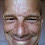Back when Nixon was President, I passed calculus. But now...but I trust John Cochrane. But I will ask this: would a few years of moderate inflation, say in the 5% range, matter if real growth is also sustained? Also, can the Federal Reserve simply hold on to its balance sheet and rollover on it in perpetuity? This would alleviate concerns about the debt being repaid (as interest payments on the debt held by the central bank flow back into the treasury). 1.So Putin can stay in power a little longer, finish the job in the Ukraine, and storm through Poland? 2.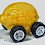Benjamin Cole, I think this is a great question. But I feel like we underestimate how much people hate inflation, is 5% truly moderate? We can judge this based, I think, on regime change, (through votes or otherwise) and civil unrest. If around the world we see lots of change I take that as 5% is not ok. Lots of new monetarist models suggest people are much more sensitive to inflation than I’ve seen a lot of mainstream economists believe. 3.Well, I lived through the 1970s. If that decade is a clue, you will soon get really good dance music, bad hair styles, and crummy automobiles. Nixon was elected in 1968-72, and then Jimmy Carter in '76. Reagan in 1980. So, politics was somewhat centrist. This time around, who knows. With housing and college bills sky-high, young people may vote for anything, and much of the employee class too. I have to say, the way to fight populism is not through scarce housing and loose labor markets. The usual de facto US policy. 2.Raising interest rates is often suggested as a way to signal the Fed's willingness to fight inflation. But in the third scenario, raising interest rates increases overall inflation vs the scenario of Fed inaction. Is the takeaway that the Fed shouldn't raise interest rates if the goal is to minimize inflation? 3.Valter Buffo. Recce'd, MilanApril 1, 2022 at 1:26 AM Useful and excellent exposition. Just one question: are more equations what we need today? Do we need to add more equations to the macro theory of the Eighties to get to the point? Still not able to understand why nobody stood up in 2017, when Trump introduced deficit-funded tax cuts? I then was answered: "we could have had an economic boom". With a 100% debt, right. And why nobody really stood up when Yellen pronunced "We have to go big" and "We can have more debt than we thought". It was disturbing to hear those words then, and still is deeply disturbing today. Even frightening. Why no reactions? In my opinion, the problem we have to solve today is mostly in the minds. Did we all for a few years adhere (some unawarely) to MMT? And, a second question: who will pay for the wrong and damaging decisions taken? And how? Nobody, and in no way. 1.Valter, "And why nobody really stood up when Yellen pronounced - 'We have to go big' and 'We can have more debt than we thought'." And now Yellen is living with those consequences: https://fred.stlouisfed.org/series/CPIAUCSL Nobody bothered to tell Yellen that the federal government (Treasury) can sell equity in lieu of bonds / debt. https://musingsandrumblings.blogspot.com/2019/09/the-case-for-equity-sold-by-u.html 4.With a combination of equity sold by the US Treasury (thereby reducing federal debt) and interest rate increases by the Federal Reserve - gold and oil prices fall, the dollar rallies, and Putin's war is brought to a screeching halt. But I am afraid there are too many vested interests in Congress on both sides of the aisle that don't want that to happen. That was what was so appealing about Ronald Reagan. He didn't have any close ties to Congressional leadership and was willing to buck the trend. I thought we might get that with Donald Trump, but he was too self involved and ignorant about making long term economic policy to have any meaningful impact. 5.Two oil producing firms - one in the US, the other in Russia. US Treasury sells non-transferrable equity to the oil producing firm in the US. Dollar rallies, oil prices crash. Firm in the US (because of it's equity) remains in business because it is more valuable as going concern than if it were liquidated in bankruptcy. See George Akerlof (husband of Janet Yellen) and his paper (along with Paul Romer) on bankruptcy for profit: https://papers.ssrn.com/sol3/papers.cfm?abstract_id=227162 Firm in Russia sees declining revenue and Russian government by extension sees declining revenue. Ruble collapses. War with Ukraine is over. 1.This comment has been removed by the author. 2.Worked for Einstein with special relativity (and a lot of help on the math side from Lorentz). 6.I'm assuming that's Matlab code since I haven't touched that in years. 7.It is good to see analysis of the problem, but Economics is much simpler than that. 1) Money added to the economy causes inflation. 2) Trillions have been authorized but not yet spent. 3) When those trillions are added to the economy, they will continue to exacerbate inflation. 4) Additional monies are being proposed and voted on as we speak; these items (increased official budget, additional spending bills, nutball ideas like400 gas cards) will continue to make inflation worse, for years.
5) Because of these facts, nothing that is done today or in the near future (such as rate hikes) will be able to stop the inflation that has already been committed.
Therefore, inflation will continue to accelerate for years, and it won't make much difference who is at the helm of this Titanic.

1.Economics is as complicated as the specifics of your question. Ask: how many years? and how much inflation?

8.The link is wrong, "the original.":

https://johnhcochrane.blogspot.com/2022/03/will-inflation-persist.html"

9.How are treating the error terms, delta.xt, delta.pit, and delta.qt ?

Have you assumed that the conditional expectations of delta.xt, delta.pit, and delta.qt are identically zero? If so, why? And if not then how do those assumptions determine the response to the disturbances driving the model output?

Viz., If, x.(t+1) = E{x.(t+1); I(t)} + delta.x(t+1), then to avoid a trivial solution the conditional expectation should be: E{delta.x(t+1); I(t)} <> 0. The delta.xt, delta.pit, and delta.qt variables in the computer program are not apparent in the listing shown. What names are associated with these stochastic variables in the program?

The quotient "primary_surplus:bond_debt_outstanding" appears to be an exogenous variable in the model. What assumptions are you making for the behavior of that variable? From the discussion, the variable seems to be an impulse, i.e., {Kronecker delta (t) x s(t) | t =0}, or a series of impulses, but that isn't clear from the computer listing (or, have I missed it?).

The 3-equation new-Keynesian DSGE model is a standard workhorse in academic circles but it performs poorly as a predictive model for real world forecasting according to David Romer, "Advanced Macroeconomics", 5 ed., 2019, NY, NY, McGraw-Hill Education. How much confidence should we be placing on the model's predictions of the price level dynamics and the central bank responses to inflation?

1.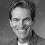Yes, E_t delta_{t+1} = 0. These derive from the behavioral equations of the model. If you start with i_t = E_t pi_{t+1}, then pi_{t+1} = i_t + delta_{t+1} with E_t delta_{t+1}=0

I'm computing the impulse response to a single shock to the surplus. So for this model, surplus is exogenous; it takes a single unexpected shock -1; and then returns to zero forever. In FTPL, I show how to make the surplus endogenous, respond to all sorts of things, but this is a blog post so I kept it really simple.

You are exactly right that the 3 equation model has lots of empirical problems. You should not take this model -- especially with made up parameters -- as a quantitative forecast! But the mode is simple, and illustrates mechanisms at work in more complex models. Always start simple, understand the mechanisms, and then layer on more model ingredients. Black box macroeconomics is pretty useless as it is so dependent on minor modeling assumptions. The point of this little model is to see how a very simple and standard form of price stickiness and a monetary rule can stretch out the "transitory" or one time price level rise of a fiscal shock to a long-lasting inflation. That mechanism is likely to be present in models of this basic form that add ingredients. Number one, as I suggested, is a lagged inflation term in the Phillips curve.

Comments are welcome. Keep it short, polite, and on topic.

Thanks to a few abusers I am now moderating comments. I welcome thoughtful disagreement. I will block comments with insulting or abusive language. I'm also blocking totally inane comments. Try to make some sense. I am much more likely to allow critical comments if you have the honesty and courage to use your real name.